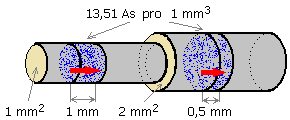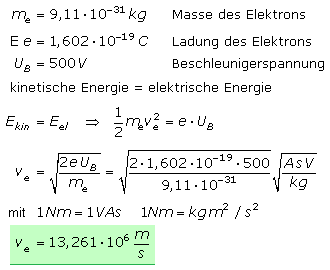# The directional drift takes place at what speed

## Electrical current

In the atomic lattice of metals, the valence and conductivity bands overlap, so that the valence electrons are freely movable in the lattice and visually behave like an electron gas. They drift around in disorderly motion in the crystal structure. In addition, the metal atoms swing around their position of rest at their lattice positions. The supply of heat increases the oscillation amplitudes and the individual atoms require more space. The metal expands. The process is reversible, because the volume decreases again when it cools down.

If an electrically neutral metal wire is connected to a voltage source, the electric field force acts on the disordered movement of the electrons. The electrons adopt an orderly, directed movement that leads to an electrical current in the conductor.

Electric voltage is the cause of electric current.
Electric current is the directional movement of charge carriers.
The current strength is the measure of the charge transport per second at any point on the conductor.

In the metallic conductor, the electric current only transports charge carriers and does not change the material. The mechanical interaction of the drifting electrons with other electrons and metal atoms in the solid lattice can cause heating. The rise in temperature increases the thermal oscillations of the particles and thereby reduces the mean free path of the free electrons. The directed flow of current is hindered and decreases. The symbol for electric current is I. The unit of measurement is the ampere with the abbreviation A.

### Definition of the amperage

In a vacuum, two ideal conductors of infinite length with a negligibly small diameter are arranged at a distance of 1 m from one another. If a temporally constant current of 1 A flows through these lines, the force 2 · 10exp − 7 N is exerted on each conductor element 1 m in length. This definition eludes measurement technology.

The mass of the electrodeposited silver is determined according to the historical metrological method. An electrical current of 1 A constant over time separates 1.118 mg of silver from an aqueous silver nitrate solution as a result of electrolysis in one second.

According to the new definition, electrical current transports electrical charges and is defined as the amount of charge per unit of time. 1 A = 1 C / s, where 1 C = 6.24151 · 10exp18 correspond to elementary charges. The elementary charge of the electron is given as e = 1.6021 · 10exp − 19 As = C. The linked article describes the current definitions of the SI units.

### Electron conduction

Only electrons are responsible for conducting electrical current in metals. Since the elementary charge of an electron is very small, the charge transport of extremely many electrons per second (≈6.24 · 10exp18 C / s) is necessary for a current of 1 ampere. In the metallic conductor, the drift speed of an electron is only a few millimeters per second after an electric field is applied. After switching on, the current flows immediately at every point in the circuit. So that the conductor remains electrically neutral, an electron entering at the beginning of the conductor forces another electron to leave the end of the conductor almost at the same time. The movement impulse or the information about the electric shock propagates in the electric circuit at almost the speed of light, around 300,000 km / s. Depending on the conductor material and its structure, this information transport can be up to 30% slower.

### Ionic conduction

In electrolyte solutions or molten salts, the charge transport takes place through differently charged ions. An electrolyte is also neutral to the outside world. Some ions can transport more than one elementary charge. Compared to electrons, ions are larger and their drift speed is slower than that of the free electrons in the metal. In addition to the temperature, the speed of the ions is particularly dependent on the concentration and degree of dissociation of the electrolyte. A charge exchange takes place at the electrodes with the electrons flowing in the external circuit.

### Power line in gases

A power line is also possible in gases and in a real vacuum (diluted gases). In gases, both electrons and ions of the excited gas molecules are involved in charge transport. The temperature increase caused by the current can increase the kinetic energy of the particles so that when they collide with neutral gas molecules, new charge carriers are generated and the current flow increases.

### Power line in vacuum tubes

In the vacuum tube, the flow of current is caused by electrons. They emerge from the cathode through thermal excitation of the filament or other suitable substances. This process is called thermal emission. The electrons then move to the positive pole of the electron tube and close the circuit to the voltage source via the anode contact.

Negative charge carriers move from the negative pole to the positive pole.
Positive charge carriers move from the positive pole to the negative pole.
The physical direction of the current is defined as the flow of electrons from the cathode to the anode.
The conventional or technical direction of current is set from plus to minus.

Not so scientifically, but with the joke on the neck, the current can be explained understandably even for a non-expert.

### Electron speed in the metallic conductor

The cause of the electric current is directed movements of charge carriers. In most cases it is the electrons. In metallic conductors, these are the only movable charge carriers. For each current value, the speed, known as the drift speed, can be calculated in the conductor material.

As the current I increases, more electrons have to be moved through the conductor per unit of time. An electrically neutral conductor only provides a certain number of electrons. The more current is supposed to flow, the faster the electrons have to move through the conductor. At constant temperature, constant cross-sectional area and constant charge density, the drift velocity v is directly proportional to the electric current: v ~ I.

There are more charge carriers in a larger cross-sectional area A and a lower electron speed is sufficient for the same amount of current per unit of time. The drift speed is inversely proportional to the area: v ~ 1 / A.

If a conductor could provide more charge carriers, the same current value would be achieved at a constant temperature and the same cross-sectional area with a lower electron speed. The drift speed is therefore inversely proportional to the charge density: v ~ 1 / ρq.

The charge density is the number of charge carriers e based on a volume element. ρq = n e / V. Physical chemistry says that 1 mole of a substance always consists of 6.02205 · 1023 Particle consists. This number is known as the Avogadro number. Molecular weight is the molecular weight in grams and contains exactly this number of particles. The charge carrier density is equal to the number of particles named mol−1 (Avogadro number) multiplied by the density of the conductor material in kg / m³ and divided by its molecular mass in kg / mol.

The drift speed of the electrons depends on the conductor material, its cross-section, the current strength and the temperature. With increasing temperature, the Brownian molecular motion increases and shortens the mean free path of the electrons. They often get caught in the repulsive electric fields of neighboring electrons, as a result of which their relative speed decreases. The influence of temperature is not taken into account in the following calculations. The mean drift speed of the electrons in the copper wire is to be calculated. The wire has a cross section of 1 mm2. The current is 1 A.

Each copper atom provides 1 electron to conduct electricity.
The molar mass of copper is 63.6 g / mol.
1 mole contains 6.022 x 1023 Atoms.
Thus, 1 mole of copper (63.6 g) represents 6.022 x 1023 Electrons available.
The density of copper is around 8.93 mg / mm3, it follows:
1 mm3 Copper corresponds to 0.14 x 10−3 mol. Multiplied by the number of electrons / mole that means:
1 mm3 Copper yields 8.43 x 1019 Electrons to conduct electricity.
Each electron carries the elementary charge of 1.6022 · 10−19 A · s = C (coulomb).
1 mm3 Copper provides 13.51 A · s to the power line.

Electric current means charge transport per unit of time. If 13.51 A · s is transported by 1 mm / s, 13.51 A will flow. The required current flow should be 1 A. In this example, the electrons move very slowly through the conductor at v = 0.074 mm / s.If the cross-section of the copper wire is increased, the ohmic resistance is reduced proportionally. The speed of the electrons in the conductor decreases. With a wire cross-section of 2 mm2 and the intended current of 1 A, half the distance is required. The electron speed is halved.

The lower the ohmic resistance of a metallic conductor, the lower the relative speed of the electrons when conducting electricity.

### The electron speed in a vacuum

In vacuum electron tubes, the flow of current is also based on electrons. They are generated by a glow electrode, the cathode, and accelerated towards the anode by a positive anode voltage. To enable this process, the tube must be well evacuated. The speed of the electrons depends on the anode voltage. The higher it is, the greater the force on the individual electron. It is accelerated more strongly and its speed increases.

The following example calculates the speed of electrons in a vacuum tube. The acceleration voltage is so small that the relativistic increase in mass does not yet have to be taken into account.Physics teaches that the particle mass increases at very high speeds. In relativistic physics, the speed of light is c = 2.99792 · 108 m / s a ​​universal natural constant. The relativistic increase in mass is derived from this. These prerequisites must always be taken into account when the acceleration energy is large compared to the rest energy of the accelerated particle. The rest energy of the electron is: me · c2 = 0.511 MeV = 8.2 x 10−14 N · m. The derivation uses m for the relativistic electron mass and me for the electron mass with the assumption that the total energy is the sum of the kinetic energy and the rest energy of the electron.The acceleration voltage should now be 25 kV. It is therefore in the range of the anode voltage of a monitor picture tube (CRT). The speed of the electrons calculated according to the simplified relationship gives: v = 93.769 · 106 m / s. If the relativistic increase in mass is taken into account, the value is calculated as follows: v = 90.482 · 106 m / s. The electrons move much more slowly.